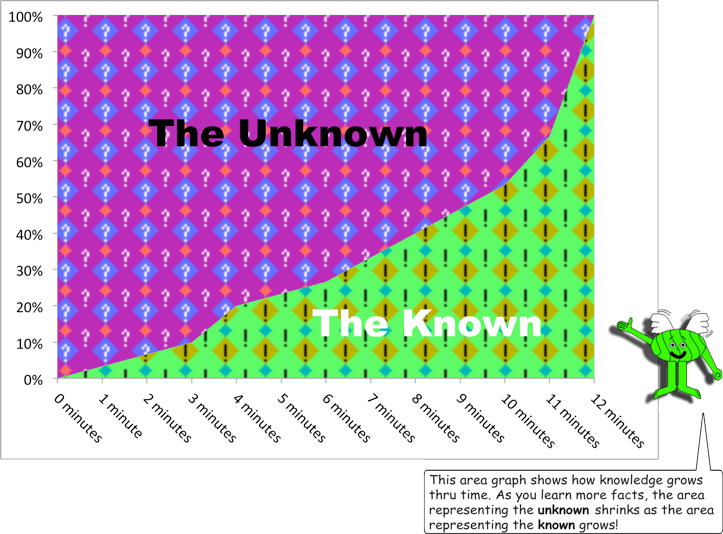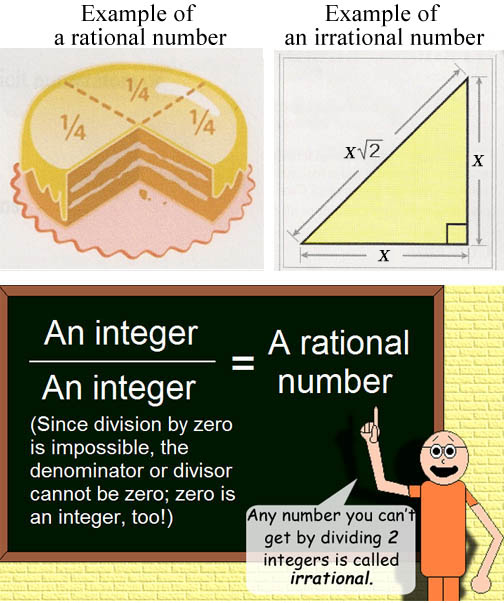# Knowledge Vs. Mystery## Knowledge = Progress * Time

(For example: If you learn 5 facts per second in 1 minute(or 60 seconds), then you will learn 300 facts!) Steeper slopes on the graph above suggest a speed boost in learning more facts!

Mystery is the opposite of knowledge since mystery is based on the unknown. When mysteries are solved, they become known facts. A fact is a piece of knowledge!

Sometimes, people may forget what they learned, but hopefully something will refresh their memory! Forgetting seems to undo learning; in fact, the 2 verbs could be opposites. Anyway, if you know how to do math well, you can use it to refresh your memory, especially when considering calculations & numerical data.

## Here's a learning strategy: If you don't know a fact, then know how to find out!

Before I conclude this Web page, I want to mention something important when comes to measuring things, like time, length & so on. A quantity regarded as a whole in calculation is called a unit. Smaller units can be fractions of bigger units! (Like how 12 inches = 1 foot. It's quite interesting that there's a unit of length named after a body part!) To convert bigger units to smaller units, multiply by the total number of smaller units in the bigger unit. To convert smaller units to bigger units, divide by the total number of smaller units in the bigger unit.

Finally, here's another reason why irrational numbers are irrational: They have no measure in common with the unit! I'm referring the smallest natural number: 1. To mention a specific irrational number, the square root of 2 in length, considering an inch for example, has no measure in common with a single inch. The Pythagorean theorem is a coincidence, but it's a wonderful coincidence!